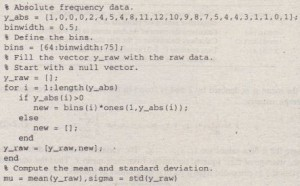Gauss Elimination

Statistical analysis of data on human proportions is required in many engineering applications. For example, designers of submarine crew quarters need to know how small they can make bunk lengths without eliminating a large percentage of prospective crew members. Use MATLAB to estimate the mean and standard deviation for the height data given in Table 7.2-1.

Solution

The script file follows. The data given in Table 7.2-1 is the absolute frequency data and is stored in the vector y..:..abs. A bin width of 1/2 in. is used because the heights were measured to the nearest 1/2 in. The vector bins contains the heights in 1/2 in. increments. Te.compute the mean and standard deviation, reconstruct the original (raw) height’ data from the absolute frequency data. Note that this data has some zero entries. For example, none of the 100 men had a height of 6S in. Thus to reconstruct the raw data, start with a null vector y_raw and fill it with the height data obtained from the absolute frequencies. The for loop checks to see whether the absolute frequency for a particular bin is nonzero. If it is nonzero, append the appropriate number of data values to the vector y_raw. If the particular bin frequency is 0, y_raw is left unchanged.When you run this program, you will find that the mean is J-L = 69.6 in. and the standard deviation is (1 = 1.96 in.